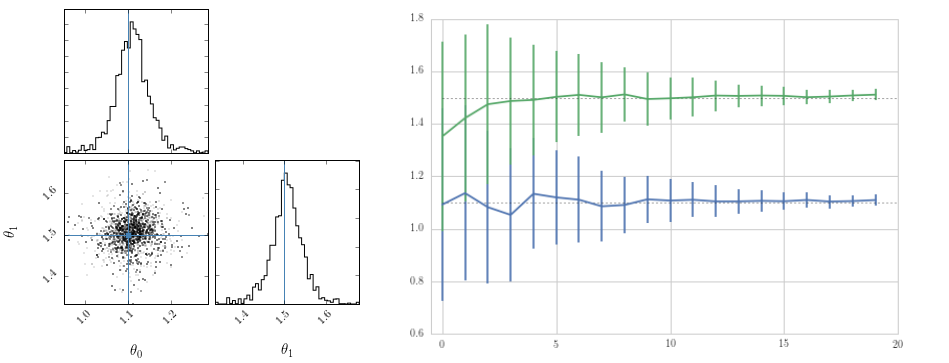# abcpmc¶A Python Approximate Bayesian Computing (ABC) Population Monte Carlo (PMC) implementation based on Sequential Monte Carlo (SMC) with Particle Filtering techniques.The abcpmc package has been developed at ETH Zurich in the Software Lab of the Cosmology Research Group of the ETH Institute of Astronomy.

The development is coordinated on GitHub and contributions are welcome. The documentation of abcpmc is available at readthedocs.org and the package is distributed over PyPI.

## Features¶

• Entirely implemented in Python and easy to extend

• Follows Beaumont et al. 2009 PMC algorithm

• Parallelized with muliprocessing or message passing interface (MPI)

• Extendable with k-nearest neighbour (KNN) or optimal local covariance matrix (OLCM) pertubation kernels (Fillipi et al. 2012)

• Detailed examples in IPython notebooks

### Contents:¶

#### Installation¶

At the command line via pip:



#### abcpmc Package¶

##### sampler Module¶
class abcpmc.sampler.Sampler(N, Y, postfn, dist, threads=1, pool=None)[source]

ABC population monte carlo sampler

Parameters: N – number of particles Y – observed data set postfn – model function (a callable), which creates a new dataset x for a given theta dist – distance function rho(X, Y) (a callable) threads – (optional) number of threads. If >1 and no pool is given multiprocesses will be started pool – (optional) a pool instance which has a function
close()[source]

Tries to close the pool (avoid hanging threads)

particle_proposal_cls

alias of ParticleProposal

sample(prior, eps_proposal, pool=None)[source]

Launches the sampling process. Yields the intermediate results per iteration.

Parameters: prior – instance of a prior definition (or an other callable) see sampler.GaussianPrior eps_proposal – an instance of a threshold proposal (or an other callable) see sampler.ConstEps pool – (optional) a PoolSpec instance,if not None the initial rejection sampling

will be skipped and the pool is used for the further sampling

Yields pool: yields a namedtuple representing the values of one iteration
class abcpmc.sampler.GaussianPrior(mu, sigma)[source]

Normal gaussian prior

Parameters: mu – scalar or vector of means sigma – scalar variance or covariance matrix
class abcpmc.sampler.TophatPrior(min, max)[source]

Tophat prior

Parameters: min – scalar or array of min values max – scalar or array of max values
class abcpmc.sampler.ParticleProposal(sampler, eps, pool, kwargs)[source]

Creates new particles using twice the weighted covariance matrix (Beaumont et al. 2009)

class abcpmc.sampler.OLCMParticleProposal(sampler, eps, pool, kwargs)[source]

Creates new particles using an optimal loacl covariance matrix (Fillipi et al. 2012)

class abcpmc.sampler.KNNParticleProposal(sampler, eps, pool, kwargs)[source]

Creates new particles using a covariance matrix from the K-nearest neighbours (Fillipi et al. 2012) Set k as key-word arguement in abcpmc.Sampler.particle_proposal_kwargs

abcpmc.sampler.weighted_cov(values, weights)[source]

Computes a weighted covariance matrix

Parameters: values – the array of values weights – array of weights for each entry of the values the weighted covariance matrix
abcpmc.sampler.weighted_avg_and_std(values, weights, axis=None)[source]

Return the weighted avg and standard deviation.

Parameters: Returns avg, sigma: values – Array with the values weights – Array with the same shape as values containing the weights axis – (optional) the axis to be used for the computation weighted average and standard deviation
##### threshold Module¶

Various different threshold implementations

Created on Jan 19, 2015

author: jakeret

class abcpmc.threshold.ConstEps(T, eps)[source]

Constant threshold. Can be used to apply alpha-percentile threshold decrease :param eps: epsilon value

class abcpmc.threshold.EpsProposal(T)[source]

Bases: object

next()[source]
reset()[source]
class abcpmc.threshold.ExponentialConstEps(max, min, T1, T2)[source]
class abcpmc.threshold.ExponentialEps(T, max, min)[source]

Exponentially decreasing threshold

Parameters: max – epsilon at t=0 min – epsilon at t=T T – number of iterations
class abcpmc.threshold.LinearConstEps(max, min, T1, T2)[source]

Linearly decreasing threshold until T1, then constant until T2

Parameters: max – epsilon at t=0 min – epsilon at t=T T1 – number of iterations for decrease T2 – number of iterations for constant behavior
class abcpmc.threshold.LinearEps(T, max, min)[source]

Linearly decreasing threshold

Parameters: max – epsilon at t=0 min – epsilon at t=T T – number of iterations
class abcpmc.threshold.ListEps(T, eps_vals)[source]
##### mpi_util Module¶

A helper util for message passing interface (MPI) handlings

class abcpmc.mpi_util.MpiPool(mapFunction=<built-in function map>)[source]

Bases: object

isMaster()[source]

Returns true if the rank is 0

map(function, sequence)[source]
abcpmc.mpi_util.mpiBCast(value)[source]

Mpi bcasts the value and returns the value from the master (rank = 0).

#### Contributing¶

Contributions are welcome, and they are greatly appreciated! Every little bit helps, and credit will always be given.

You can contribute in many ways:

##### Types of Contributions¶
###### Report Bugs¶

If you are reporting a bug, please include:

• Your operating system name and version.
• Detailed steps to reproduce the bug.
###### Write Documentation¶

abcpmc could always use more documentation, whether as part of the official abcpmc docs, in docstrings, or even on the web in blog posts, articles, and such.

###### Submit Feedback¶

If you are proposing a feature:

• Explain in detail how it would work.
• Keep the scope as narrow as possible, to make it easier to implement.
• Remember that this is a volunteer-driven project, and that contributions are welcome :)
##### Pull Request Guidelines¶

Before you submit a pull request, check that it meets these guidelines:

1. The pull request should include tests.
2. If the pull request adds functionality, the docs should be updated. Put your new functionality into a function with a docstring, and add the feature to the list in README.rst.
3. The pull request should work for Python 2.6, 2.7, and 3.3, and for PyPy. make sure that the tests pass for all supported Python versions.
##### Tips¶

To run a subset of tests:

\$ py.test test/test_abcpmc.py